出租广告位,需要合作请联系站长

+关注

2020-07(10)

2020-08(50)

# 一、Numpy

NumPy 的主要对象是齐次多维数组。它是一个元素表（通常是元素是数字），其中所有元素类型都相同，元素以正整数元组索引。在 NumPy 维度（dimension）被称为轴（axis）。

ps. 有几个轴就是几维数组，符合平时生活中有 x, y 两个坐标轴就是二维空间，再加上 z 轴就是三维空间的概念

``````[[ 1., 0., 0.],
[ 0., 1., 2.]]
``````

Numpy 的数组类称做 ndarry，别名是 array。注意 numpy.array 和 Python 标准库的类 array.array 不同，标准库的类只处理一维数组（one-dimensional arrays）。

## 1. 重要属性

• ndarray.ndim

the number of axes (dimensions) of the array.

• ndarray.shape

数组的维度（the dimensions of the array）。 以一个整型元组的方式表示数组中每个维度的大小。比如对一个有 n 行 m 列的矩阵来说，其 shape 属性为 (n, m)。The length of the shape tuple is therefore the number of axes, ndim.

• ndarray.size

数组元素总数。相当于 shape 中每个元素的乘积。

• ndarray.dtype

一个用来描述数组中元素类型的对象。我们可以使用 Python 标准类型来创建指定该对象，NumPy 也提供了自己的类型，如 numpy.int32, numpy.int16, and numpy.float641 等

• ndarray.itemsize

数组中每个元素的字节大小。 For example, an array of elements of type float64 has itemsize 8 (=64/8), while one of type complex32 has itemsize 4 (=32/8). It is equivalent to ndarray.dtype.itemsize.

# 二、Matpltlib

Matplotlib是python的绘图库，经常与Numpy一起使用，停工了有效的Matlab开源替代方案，也可以和图形工具包一起使用，比如PyQt和wxPython。

``````plt.title("图标题")
plt.xlabel("x轴标识")
plt.ylabel("y轴标识")
``````

``````x = np.arange(1,11)
y =  2  * x +  5
``````

## 1.Plot()

``````plt.plot(x,y)
plt.show()
``````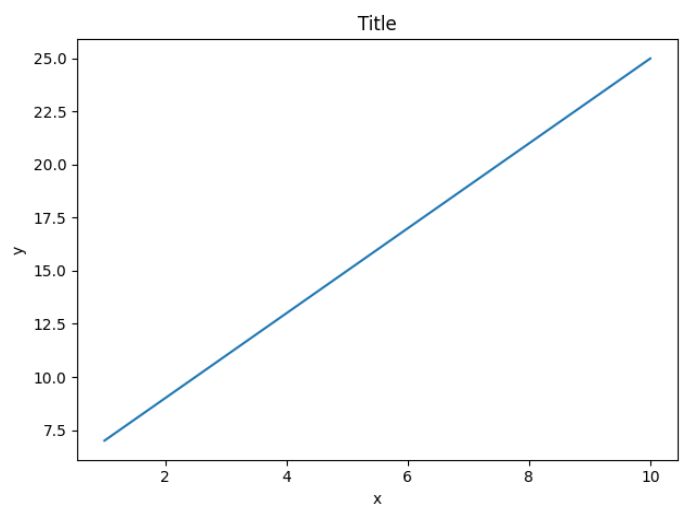``````plt.plot(x,y,"ob")
plt.show()
``````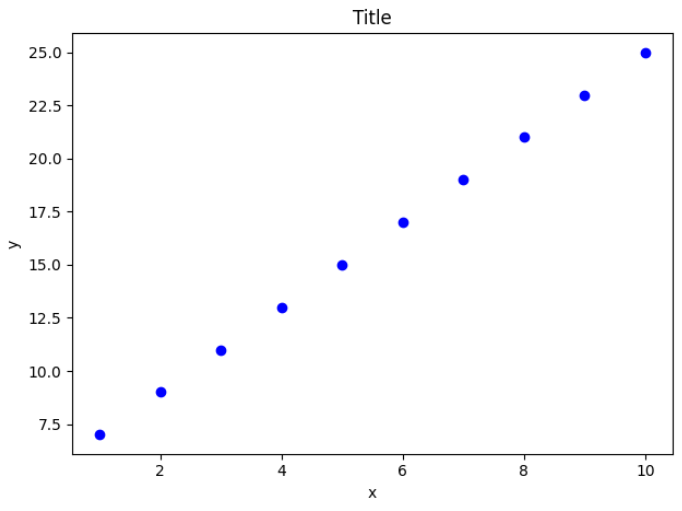## 2.Subplot()

``````import numpy as np
import matplotlib.pyplot as plt
# 计算正弦和余弦曲线上的点的 x 和 y 坐标
x = np.arange(0,  3  * np.pi,  0.1)
y_sin = np.sin(x)
y_cos = np.cos(x)
# 建立 subplot 网格，高为 2，宽为 1
# 激活第一个 subplot
plt.subplot(2,  1,  1)
# 绘制第一个图像
plt.plot(x, y_sin)
plt.title('Sine')
# 将第二个 subplot 激活，并绘制第二个图像
plt.subplot(2,  1,  2)
plt.plot(x, y_cos)
plt.title('Cosine')
# 展示图像
plt.show()
``````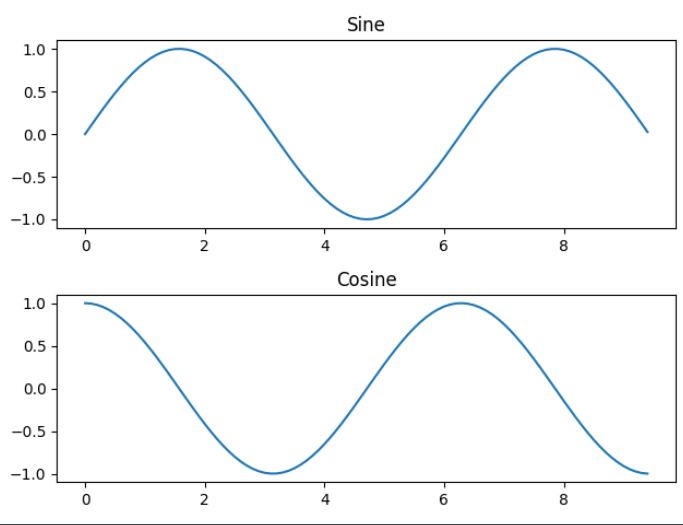## 3.Bar()

pyplot子模块提供bar()来生成条形图。

``````from matplotlib import pyplot as plt
x =  [5,8,10]
y =  [12,16,6]
x2 =  [6,9,11]
y2 =  [6,15,7]
plt.bar(x, y, align =  'center')
plt.bar(x2, y2, color =  'g', align =  'center')
plt.title('Bar graph')
plt.ylabel('Y axis')
plt.xlabel('X axis')
plt.show()
``````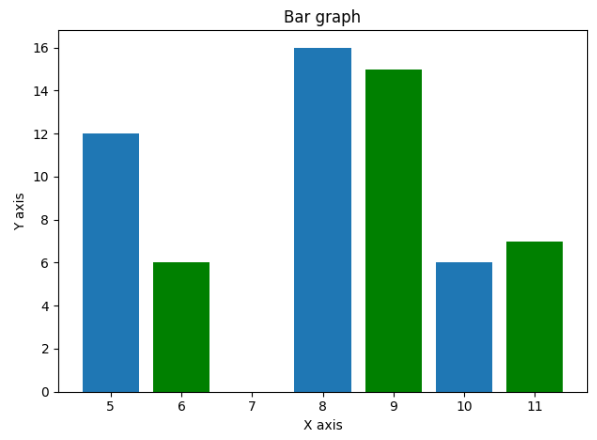## 4.Numpy.histogram()

numpy.histogram()函数将输入数组和 bin 作为两个参数。 bin 数组中的连续元素用作每个 bin 的边界。

``````a = np.array([22,87,5,43,56,73,55,54,11,20,51,5,79,31,27])
hist,bins = np.histogram(a,bins =  [0,10,20,30,40,100])
print (hist)
print (bins)
``````

``````[2 1 3 1 8]
[  0  10  20  30  40 100]
``````

## 5.Hist()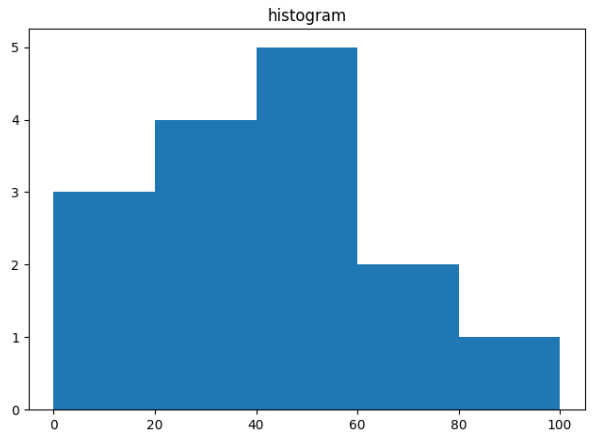### hist函数详解

#### 函数内容

``````def hist(
x, bins=None, range=None, density=False, weights=None,
cumulative=False, bottom=None, histtype='bar', align='mid',
orientation='vertical', rwidth=None, log=False, color=None,
label=None, stacked=False, *, data=None, **kwargs):
return gca().hist(
x, bins=bins, range=range, density=density, weights=weights,
cumulative=cumulative, bottom=bottom, histtype=histtype,
align=align, orientation=orientation, rwidth=rwidth, log=log,
color=color, label=label, stacked=stacked,
**({"data": data} if data is not None else {}), **kwargs)

``````

#### 函数详解

x : (n,) array or sequence of (n,) arrays
(数组或（允许长度不等的）数组序列)

bins：整数值或序列。

• 如果bins为整数值，则bins为柱子个数，根据数据的取值范围和柱子个数bins计算每个柱子的范围值，柱宽=(x.max()-x.min())/bins。例：数据取值为[1,2,3,4,5,6]，bins=6，柱宽=0.8333…，则每个柱子的范围分别为：[1,1.83), [1.83,2.66)…，[5.17,6]
• 如果bins取值为序列，则该序列给出每个柱子的范围值（即边缘）。除最后一个柱子外，其他柱子的取值范围均为半开（左闭右开）。接上例，数据共n=6个取值，若bins=range(1,n+2)即bins的取值为[1,2,3,4,5,6,7]，注意range用法。则每个柱子的范围为：[1,2),[2,3),…,[5,6),[6,7]。注意：这里可以看出为什么bins的取值需要包含7，因为最后一个柱子的取值范围是全闭的，如果取值只到6，则最后一个柱子的范围为[5,6]，取值为5和6的数据将被合并计算。

range : tuple or None, optional

density：布尔值

normed : boolean, optional(?)是否将得到的直方图向量归一化
normed=True表示正则化直方图，即归一化（即求频率）

weights : (n, ) array_like or None, optional

cumulative : boolean, optional

bottom : array_like, scalar, or None

histtype : {‘bar’, ‘barstacked’, ‘step’, ‘stepfilled’}, optional(选择展示的类型,默认为bar)
'bar’是传统的条形直方图；'barstacked’是堆叠的条形直方图；'step’是未填充的条形直方图，只有外边框；‘stepfilled’是有填充的直方图。当histtype取值为’step’或’stepfilled’，rwidth设置失效，即不能指定柱子之间的间隔，默认连接在一起。

align : {‘left’, ‘mid’, ‘right’}, optional(对齐方式)
‘left’：柱子的中心位于bins的左边缘；‘mid’：柱子位于bins左右边缘之间；‘right’：柱子的中心位于bins的右边缘。可在上面两张图中求证。

orientation : {‘horizontal’, ‘vertical’}, optional(直方图方向)

log : boolean, optional(log刻度)

color : color or array_like of colors or None, optional(颜色设置)

label : string or None, optional(刻度标签)

stacked : boolean, optional

#### 返回的参数

frequency（官方文档用n表示）：数组或数组列表。柱子的高，即频数或频率值，数据类型为float。

bins：每个柱子的左右边缘值（即区间范围），例子见上文输入参数bins。

patches：列表或列表的列表。取值为每个柱子包含的数据。

## 直方图和条形图

• 条形图：条形图用长条形表示每一个类别，长条形的长度表示类别的频数，宽度表示表示类别。
• 直方图：直方图是一种统计报告图，形式上也是一个个的长条形，但是直方图用长条形的面积表示频数，所以长条形的高度表示 频数/组距 ，宽度表示组距，其长度和宽度均有意义。当宽度相同时，一般就用长条形长度表示频数。

# 三、平滑直方图

x是直方图平滑前各个柱子的值组成的数组

``````def Smooth_f(x):
'''
用来实现直方图数据平滑的函数
:param x: 输入一个列表
:return:
'''
print("smooth begin")
print(x)
y = x.copy()
for i in range(1, len(y)):
y[i] = 0.5*(y[i]+y[i-1])
# print([i, y[i]])
print(y)
return y
``````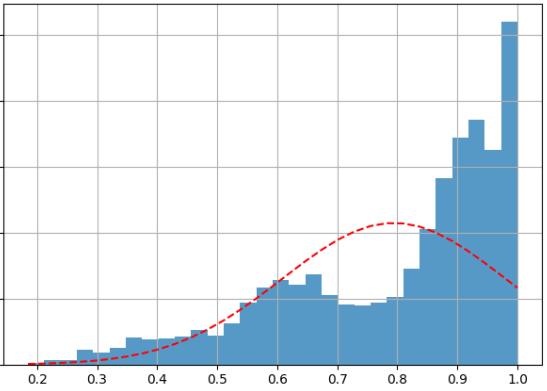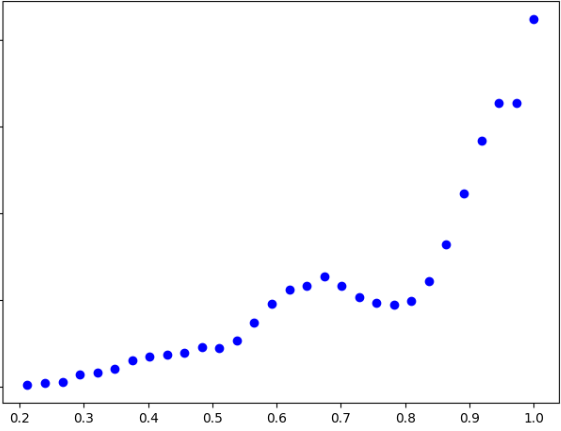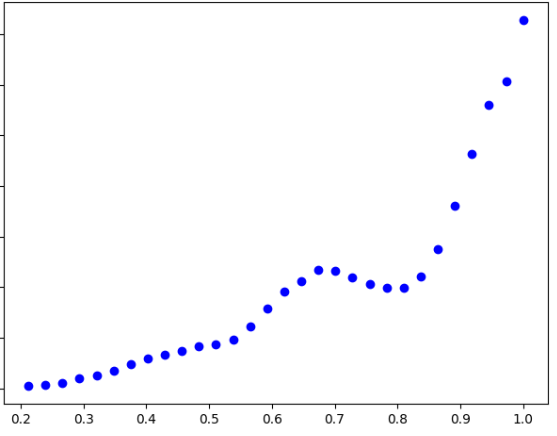7 0

pdf(new) 更多>